# Magnetic Effect of Current Formulae For NEET

When current is passed through the conductor it will produce a magnetic effect around it, so basically the wire acts like a magnet, and it will interact with the permanent magnet you have placed next to it, this effect can be reversed by changing the direction of the current, which according to the rule changes the direction of the magnetic field produced by it.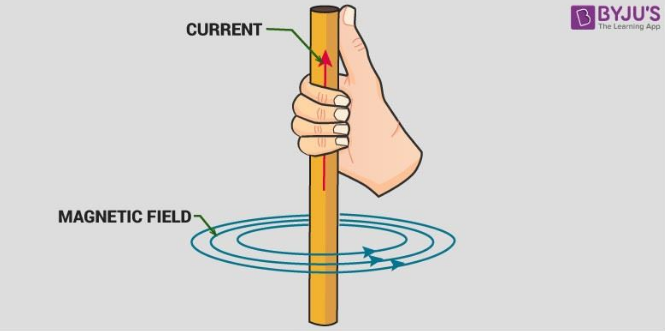1. Magnetic field due to a moving point charge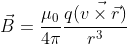μo ≡ 4π × 10-7 N·s2/C2 is called the permeability of free space

2. Biot- savart’s Law: This law states that the magnetic field (dB) at point P due to small current element Idl of the current-carrying conductor is directly proportional to the Idl (current) element of the conductor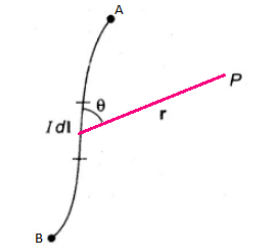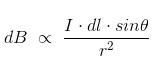3. Magnetic field due to a straight wire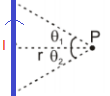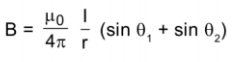4. Magnetic field due to an infinite straight line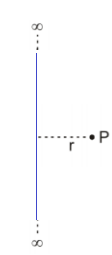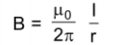5. Magnetic field due to a circular loop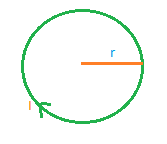i) At centre

B= μ0NI/2r

ii) At axis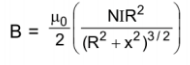6. Magnetic field on the axis of a solenoid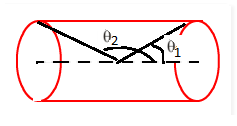B = (μ0NI/2) (cos θ1 – cos θ2)

7. Amperes Law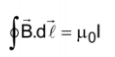8. Magnetic field due to a long cylinder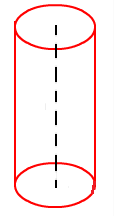i) B= 0, r ＜ R

ii) B = μ0I/2πr, r ≥ R

9. Magnetic force acting on a moving point charge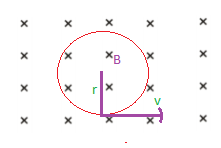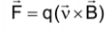10. Magnetic force acting on a current-carrying wire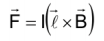11. Magnetic Moment of a current carrying loop M = NIA
12. The torque acting on a loop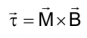13. Magnetic field due to single poleB = (μ0/2π) m/r2
14. Magnetic field on the axis of the magnetB = (μ0/4π) 2M/r3
15. Magnetic field on the equatorial axis of the magnetB = (μ0/4π) M/r3
16. Magnetic field at the point P of the magnet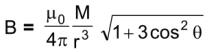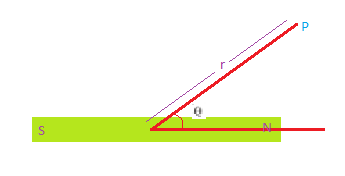Related posts: